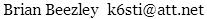# Stereo S/N Penalty

Over the years I've seen various figures quoted for the S/N difference between monophonic and stereophonic FM reception. I decided to write a short computer program to calculate the number.

```	FOR f = 20 TO 15000					' Integrate L+R and L-R noise power
d = 1 / (1 + (2 * 3.141593 * 75E-6 * f) ^ 2)		' Deemphasis filter power factor
b = b + d * f ^ 2					' L+R power
s = s + d * ((38000 - f) ^ 2 + (38000 + f) ^ 2)	' L-R power
NEXT f

PRINT 10 * LOG((b + s) / b / .91 ^ 2) / LOG(10)		' .91: 9% pilot, LOG is natural log
```

The result is 23.0 dB for a frequency range of 20 to 15000 Hz and a 9% stereo pilot. This figure should be adjusted for several nonideal effects described below. The calculated figure varies a couple tenths of a dB depending on the low-frequency limit and pilot level. For a high-frequency limit of 10000 Hz, the S/N difference becomes 25.0 dB. For 5000 Hz, it is 28.8 dB. The following curves reveal why.Above its 2122 Hz corner frequency, the 75 µs deemphasis network with its 6 dB/octave rolloff flattens the 6 dB/octave rise of monophonic noise. But stereo noise comes mostly from the L−R subcarrier region where changes in LSB and USB noise levels are roughly complementary, with little rise for the sum. This exposes the deemphasis curve and explains why stereo noise sounds lowpassy.

The calculations assume only that composite noise rises 6 dB/octave. This may not be true if the signal is very weak and near the FM detector threshold, or very strong, where internal noise sources with different spectra may override the detected noise. The calculations also assume that the composite passband is flat. This seldom, if ever, is true for receivers with analog IF filters.

Including the composite response measured for a tuner with two 250 kHz ceramic IF filters, the calculated figure for 20–15000 Hz becomes 22.3 dB. I measured the mono/stereo S/N difference for six tuners using their wide IF filters and a 200–15000 Hz audio bandpass filter. The values ranged from 21.3 to 22.9 dB with an average of 22.2 dB. The calculated figure for 200–15000 Hz is 21.9 dB. Therefore the tuners' average excess stereo noise was 0.3 dB. Adding this to the calculated figure for 20–15000 Hz yields 22.6 dB. I think this is a good practical estimate for the difference to expect between stereo and monophonic noise for analog tuners. For DSP tuners with flat digital IF filters, flat audio to 15 kHz, and noiseless stereo decoders, expect 23.0 dB.

March 31, 201288–108 MHz